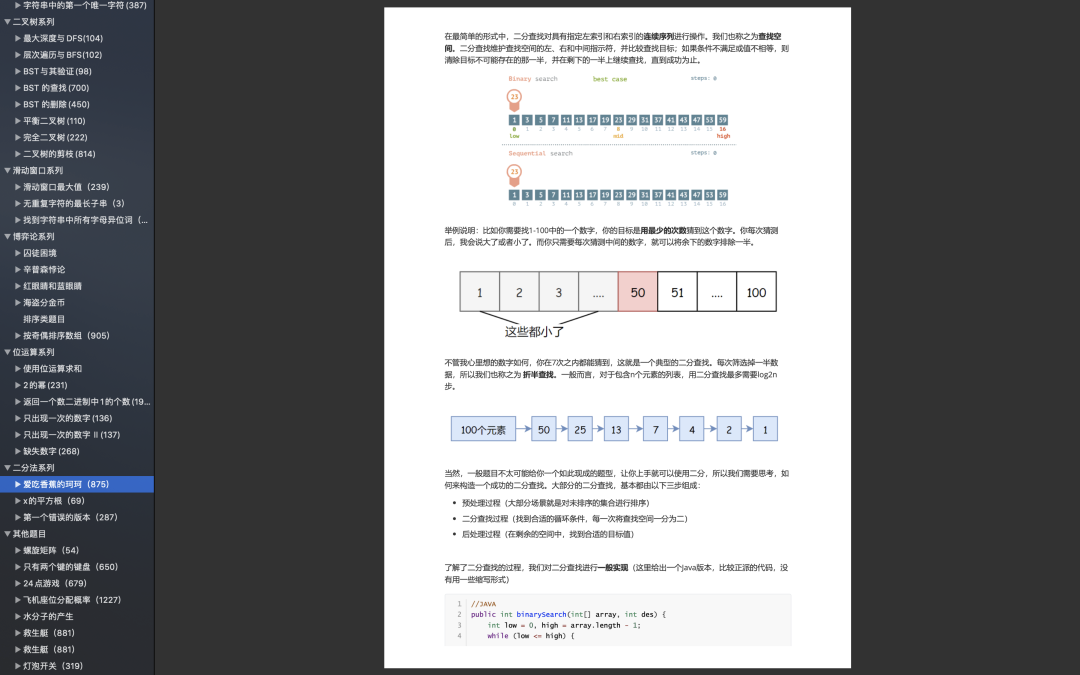## 01、题目示例

``````输入: [1,2,3,4,5]

``````输入: [0,0,1,2,5]

``````数组长度为 5

## 02、题目分析

``````//go
func isStraight(nums []int) bool {
sort.Ints(nums)
sub := 0
for i := 0; i < 4; i   {
if nums[i] == 0 {  continue  }  if nums[i] == nums[i 1] {  return false  }  sub = nums[i 1] - nums[i]  }  return sub < 5 } 复制代码``````

## 03、不排序咋整

“没吃过猪肉，也见过猪跑”这句话来源于《红楼梦》第十六回，有云：“偏你又怕他不在行了。谁都是在行的？孩子们这么大了，没吃过猪肉，也见过猪跑。”

``````//C
class Solution {
public:
bool isStraight(vector<int>& nums) {
vector<int> arr(14,0);
for(int i = 0; i < nums.size(); i){  arr[nums[i]] ;  }  for(int i = 1;i < 14; i){  if(arr[i] > 1)  return false;  }  int min = 1,max = 13;  while(min < 14 && arr[min] == 0) min ;  while(max >= 0 && arr[max] == 0) max--;  return max - min <= 4;  } }; 复制代码``````453